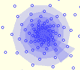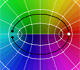Product of complex numbersWe can see it as a dilatative rotation.The multiplication by a complex number is a transformation of the complex plane: dilative rotation.From a complex number we can obtain a geometric progression obtaining the powers of natural exponent (multiplying successively) Complex FunctionsComplex power functions with natural exponent have a zero (or root) of multiplicity n in the origin.A polynomial of degree 2 has two zeros or roots. In this representation you can see Cassini ovals and a lemniscate.A complex polinomial of degree 3 has three roots or zeros.Every complex polynomial of degree n has n zeros or roots.Every complex polynomial of degree n has n zeros or roots.Podemos modificar las multiplicidades del cero y del polo de estas funciones sencillas.Una primera aproximación a estas transformaciones. Representación de dos haces coaxiales de circunferencias ortogonales.The Complex Exponential Function extends the Real Exponential Function to the complex plane.The Complex Cosine Function extends the Real Cosine Function to the complex plane. It is a periodic function that shares several properties with his real ancestor.The Complex Cosine Function maps horizontal lines to confocal ellipses.Inversion is a plane transformation that transform straight lines and circles in straight lines and circles.Inversion preserves the magnitud of angles but the sense is reversed. Orthogonal circles are mapped into orthogonal circlesThe usual definition of a function is restrictive. We may broaden the definition of a function to allow f(z) to have many differente values for a single value of z. In this case f is called a many-valued function or a multifunction.Multifunctions can have more than one branch point. In this page we can see a two-valued multifunction with two branch points. Taylor's PolynomialsWe will see how Taylor polynomials approximate the function inside its circle of convergence.The complex exponential function is periodic. His power series converges everywhere in the complex plane.The power series of the Cosine Function converges everywhere in the complex plane.